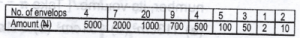# Mathematics 2019 Past Questions | JAMB

Study the following Mathematics past questions and answers for JAMBWAEC  NECO and Post-JAMB. Get prepared with official past questions and answers for upcoming examinations.

41. The diagram shows a trapezium inscribed in a semi-circle. If O is the mid-point of WZ and |WX| = |XY| = |YZ|, calculate the value of m

• A. 90 ${}^{o}$
• B. 60 ${}^{o}$
• C. 45 ${}^{o}$
• D. 30 ${}^{o}$

42.In the diagram, PQ//RS. Find x in terms of y and z

• A. x = 240$$^o$$ – y – z
• B. x = 180$$^o$$ – y – z
• C. x = 360$$^o$$ + y -z
• D. x = 360$$^o$$ – y – z

43. In the diagram, PQ is a straight line, (m + n) = 110$$^o$$ and (n + r) = 130$$^o$$ and (m + r) = 120$$^o$$. Find the ratio of m : n : r

• A. 2 : 3 : 4
• B. 3 : 4 : 5
• C. 4 : 5 : 6
• D. 5 : 6 : 7

44.Donations during the launching of a church project were sent in sealed envelopes. The table shows the distribution of the amount of money in the envelope. How much was the donation?

• A. N26,792.00
• B. N26,972.00
• C. N62.792.00
• D. N62,972.00

45A piece of thread of length 21.4cm is used to form a sector of a circle of radius 4.2cm on a piece of cloth. Calculate, correct to the nearest degree, the angle of the sector.
[Take $$\pi = \frac{22}{7}$$]

• A. 170$$^o$$
• B. 192$$^o$$
• C. 177$$^o$$
• D. 182$$^o$$

46. If tan x = $$\frac{4}{3}$$, 0$$^o$$ < x < 90$$^o$$, find the value of sin x – cos x

• A. 1\10
• B. 1\5
• C. 5\12
• D. 1 2/5

47. Given that Y is 20cm on a bearing of 300o from x, how far south of y is x?

• A. 10cm
• B. 15cm
• C. 25cm
• D. 30cm

48. The diagonals of a rhombus WXYZ intersect at M. If |MW| = 5cm and |MX| = 12cm, calculate its perimeter

• A. 42cm
• B. 48cm
• C. 52cm
• D. 60cm

49. M and N are two subsets of the universal set (U). If n(U) = 48, n(M) = 20, n(N) = 30 and n(MUN) = 40, find n(M $\cap$ N)

• A. 18
• B. 20
• C. 30
• D. 38

50.  The graph of y = x² and y = x intersect at which of these points?

• A. (0,0), (1,1)
• B. (0,0), (0,1)
• C. (1, 0), (0, 0)
• D. (0, 0) (0, 0)

## For Enquiries?

Subscribe
Notify ofInline Feedbacks